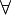# Difference between revisions of "12-240/Classnotes for Thursday October 18"

== Linear transformation ==

Definition:

A function L: V-> W is called a linear transformation if it preserve following structures:

1) L(x + y)= L(x) + L(y)

2) L(cx)= c.L(x)

3) L(0 of V) = 0 of W

Proposition:

1) property 2 => property 3

2) L: V -> W is a linear transformation iff$\forall\,\!$ c$\in\,\!$ F,$\forall\,\!$ x, y$\in\,\!$ V: L(cx + y)= cL(x) + L(y)

Proof:

1) take c= 0 in F and x=0 in V. Then L(cx)=cL(x) -> L(0 of F * 0 of V)=(0 of F)*L(0 of V)=0 of W

2)(=>)Assume L is linear transformation

L(cx + y)= L(cx) + L(y)= c*L(x) + L(y)

(<=) 1. Follows from L(c*x+y) = c*L(x)+L(y) by taking c=1

2. Follows by taking y=0

Examples

1. L: R^2 -> R^2 by

2. P,Q: P(F)